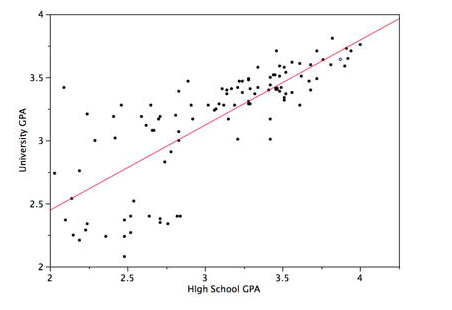# Linear Regression

Rating: 4

3979
1. Share:

## What is linear regression? Linear regression formula and example?

In this article, we will be discussing on Linear Regression process followed by an example.

Linear regression is considered to be one of the oldest and easiest regression processes that is available for everyone. This is a simple statistical tool that is still the best fit for more than 50% of all regressions.

If you would like to become an SPSS Certified professional, then visit Mindmajix - A Global online training platform:" SPSS Certification Training Course ". This course will help you to achieve excellence in this domain.

It is primarily picked up because of its simplicity and easy process.

Like all regressions, it also tries to find out a significant relationship between a dependent and independent variable.

The basic logic for the regression is to fit the present data point in a straight line such that the sum of squares of the standard deviation is minimum.

So what does linear regression line tells you?

The linear regression model attempts to convey the relationship between the two variables by giving out a linear equation to observed data. Within this, one variable is an explanatory variable (i.e. it explains something about the variable) and the other variable is marked as a dependent variable.

## Linear Regression Formula:

The formula derived is often in the form of Y= a + b * X + C where Y is the independent variable and X is the independent variable. a is the value of Y at X=0 and b is the regression proportionality constant. C, in this case, represents the value that comes from the lurking/ unknown factors.

There are various factors to be taken into consideration before doing a linear regression like

1. Presence of a linear relationship between the dependent and independent variables must be logically verified. If not then one or both the variables must be changed into functions that have a linear correlation with the other.

2. Outliers must be found out using a box plot and removed to avoid any bias in the relationship

3. Multi-collinearity needs to be dealt with before the regression is done. This is done to prevent the effect of interdependency between a different independent variable which might add to biases in the Regression analysis.

4. Heteroskedasticity must be avoided or to make it more clear the data must be normal. This is done to avoid biases and fitting the results in a straight line.It can be either simple linear regression or multiple linear regression depending on the number of independent variables. The accuracy of the model is determined by the R square factor. The assumptions must always be called out and agreed upon before the analysis. The use of linear regression is quite common in academics and industry and almost all other forms are a modification of Linear Regression model. The model stands out as the most basic and effective statistic tool ever.

Example:

A recent study which used linear regression wanted to see if there is any correlation between University GPA and high school GPA. They collected data from 100 students, removed the outliers and checked for normality in the data. Checks were done to avoid any significant collinearity and basic straight line or regression line was derived by plotting the straight line with a minimal sum of the square of standard deviation. The data and the line were created and a significant relationship between both was found. The data was converted to language by inferring the study and a causal effect for success was determined to help future generations understand the correlation by using the following results-

In this case, the Variable for taking in account the lurking factors also known as the lurking variable is found to be zero. While the constant variable a is found to be 1.097 and the regression proportionality variable is found to be 0.675.Justification of regression assumption:

The following points describe that we should assume the relationship between the variables as linear:

1. The linear relationships is one of the easiest way and simplest way to use so it helps us to image the linear relationship

2. Based on the true relationships between our variables, most of the time the relationship is approximately linear over an average.

3. Even if the variables are not in a linear format, then we can do the adjustments and bring the variables to a stage where they can show a true linear relationship.

Conclusion:

In today’s article, we talked about what is linear regression and described the formula with an example. If you think any vital points that need to be added and they are worth reading then please do key in your suggestions in the comments section below.

Related Regression Articles: